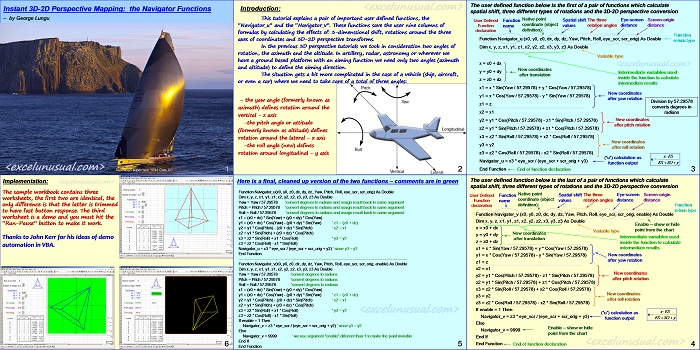# Instant 3D-2D Perspective Mapping – the Navigator Functions – a convenient pair of user defined functions

This tutorial explains a pair of important user defined functions, the “Navigator_u()” and the “Navigator_v()”. These functions save the user nine columns of formulas by calculating the effects of: 3-dimenssional shift, rotations around the three axes of coordinates and 3D-2D perspective mapping. These user defined functions are also easy to use compared to writing all the perspective mapping equations from scratch.
In the previous 3D perspective tutorials we took in consideration two angles of rotation: the azimuth and the altitude. In artillery, radar, astronomy or wherever we have a ground based platform with an aiming function, we only need two angles (azimuth and altitude) to define the aiming direction. The situation gets a bit more complicated in the case of a vehicle (ship, aircraft, or even a car) where we need to handle a total of three angles:  the yaw angle (formerly known as azimuth) defines rotation around the vertical axis, the pitch angle or attitude (formerly known as altitude) defines rotation around the lateral axis and the roll angle (new) defines rotation around longitudinal axis.

##### Thanks to John Kerr for his suggestions on automating this model in VBA. Also thanks to Fabrizio Noto for his suggestion to using a single array function instead of two simple functions in order to improve speed (see his comment below) – in this case you need to write the function =Navigator() in a certain cell, select the cell to the right (or left) and then hit F2 followed by Shift+Control+Enter (all three keys at the same time). Once we have the 2D array in one row we can then copy it down (drag)  in a regular fashion and we don’t have to use F2, Shift+Control+Enter again.[sociallocker][/sociallocker]

1.george says:

Thanks, Fabrizio. I wanted to do that exactly for the reason you mentioned (speed) since both functions almost to the end are identical but I didn’t know how to do it.

2.Fabrizio Noto says:

dear George,
why don’t you use a single UDF that returns both value (u and v).
This will increase the speed of your calculation!

Function Navigator(x0, y0, z0, dx, dy, dz, Yaw, Pitch, Roll, eye_scr, scr_orig, enable)
Dim out(1 To 1, 1 To 2) As Double

‘code shared <<<<<<<<<<<<<<<<<<<<<

out(1, 1) = x3 * eye_scr / (eye_scr + scr_orig + y2)
If enable = 1 Then
out(1, 2) = z3 * eye_scr / (eye_scr + scr_orig + y2)
Else
out(1, 2) = 9999
End If
Navigator = out
End Function

3.george says:

Bingo! You read my mind, everything will converge into a flight simulator pretty soon. Thanks for the comment. George

4.John Kerr says:

As I drove home last night, I thought you could combine this with your Virtual Joystick sheet to get the shape to spin/move in the direction of the cursor position?